# Scatter plot by group in ggplot2

## Sample data

The data below contains two numerical variables and a categorical variable representing groups. This data frame be used in the following examples.

``````set.seed(1)

# Data simulation
x <- runif(500)
y <- 5 * x ^ 2 + rnorm(length(x), sd = 2)
group <- ifelse(x < 0.4, "A",
ifelse(x > 0.8, "C", "B"))
x <- x + runif(length(x), -0.2, 0.2)

# Data frame
df <- data.frame(x = x, y = y, group = group)``````

## Scatter plot by group with `geom_point`

Creating a scatter plot by group in ggplot2 is straightforward, as you only need to pass the categorical variable to the `color` argument of `aes`.

``````# install.packages("ggplot2")
library(ggplot2)

# Scatter plot by group
ggplot(df, aes(x = x, y = y, color = group)) +
geom_point()``````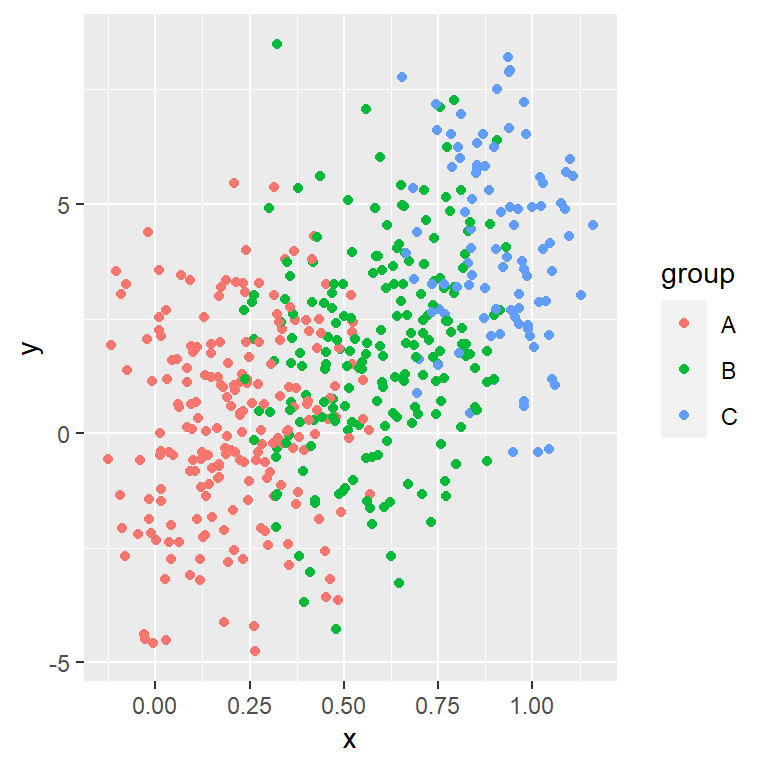Changing the colors

The default color palette can be customized in several ways. You can use `scale_color_manual` and pass a vector of colors to the `values` argument or choose a predefined discrete palette, such as `scale_color_viridis_d`.

``````# install.packages("ggplot2")
library(ggplot2)

# Vector of colors

ggplot(df, aes(x = x, y = y, color = group)) +
geom_point() +
scale_color_manual(values = cols)``````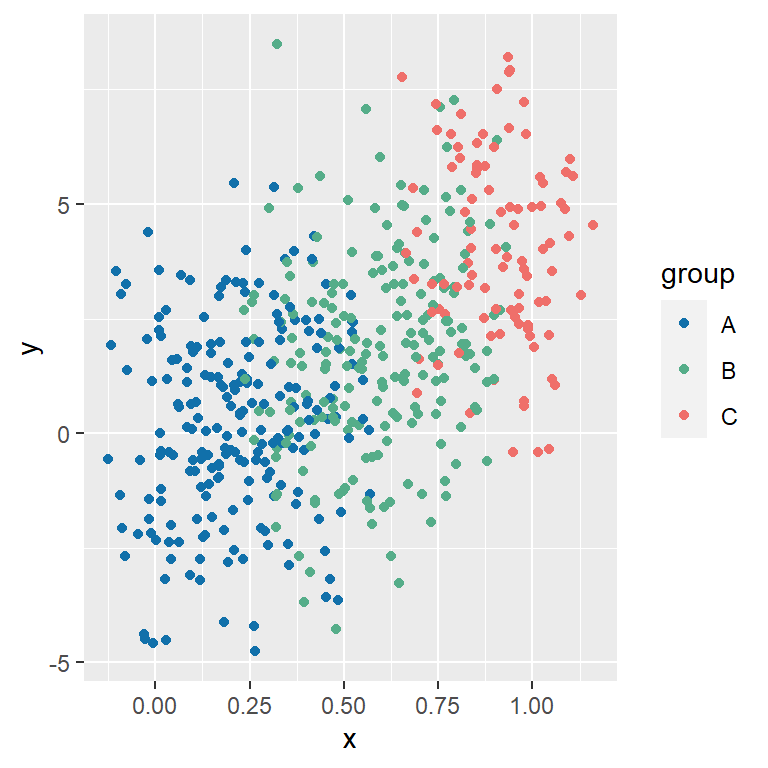Changing the shape and the size

The default shape of the observations are filled circles, but you can choose any symbol with `shape`. The size of the symbols can also be customized, making use of `size`.

``````# install.packages("ggplot2")
library(ggplot2)

ggplot(df, aes(x = x, y = y, color = group)) +
geom_point(shape = 17, size = 2)``````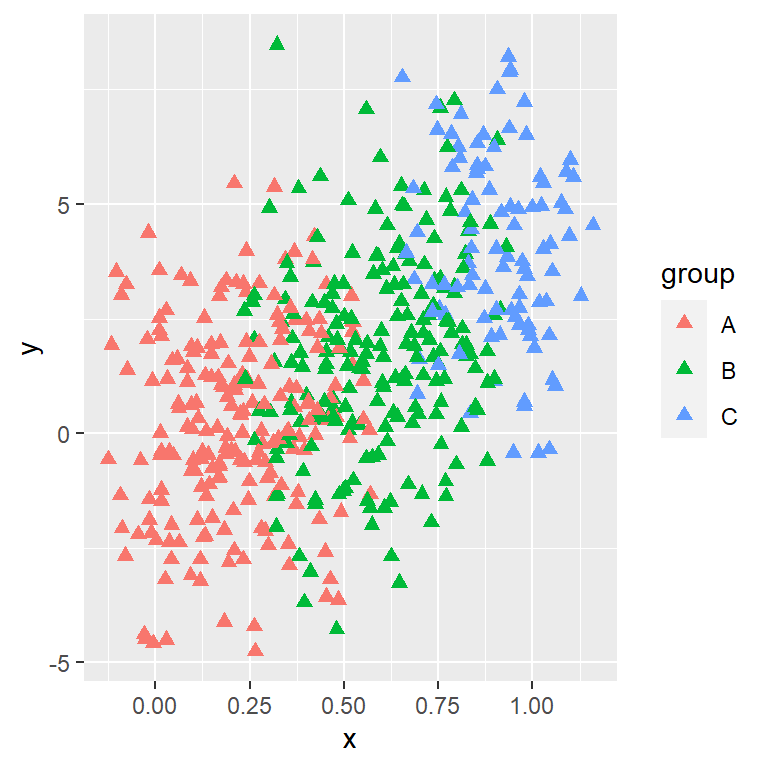Shape by group

If you want you can add a different shape by group, pass the grouping variable to the `shape` argument of the `aes` function. Note that you can specify this argument without setting `color`.

``````# install.packages("ggplot2")
library(ggplot2)

ggplot(df, aes(x = x, y = y, color = group,
shape = group)) +
geom_point(size = 2)``````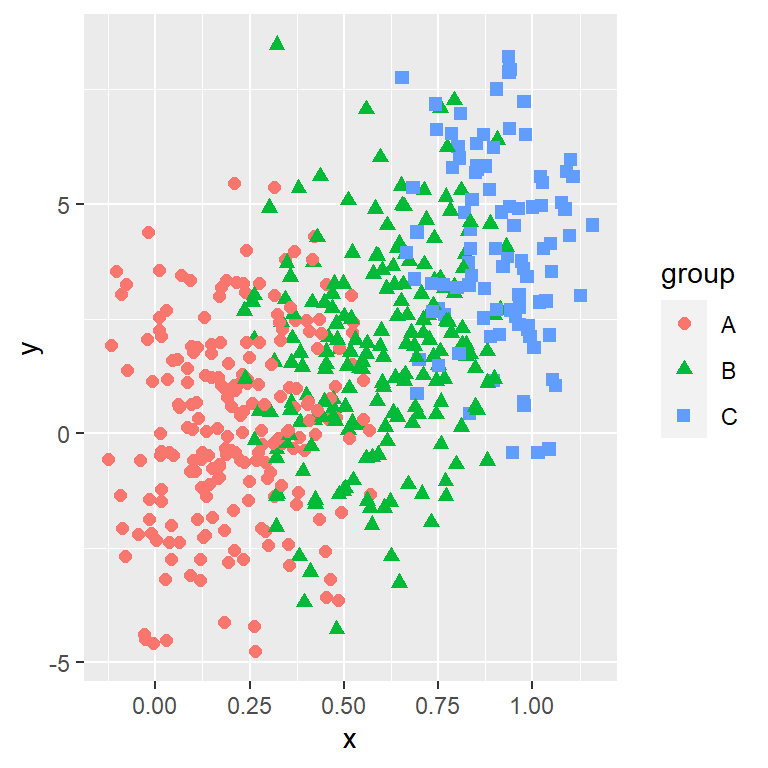## Legend customization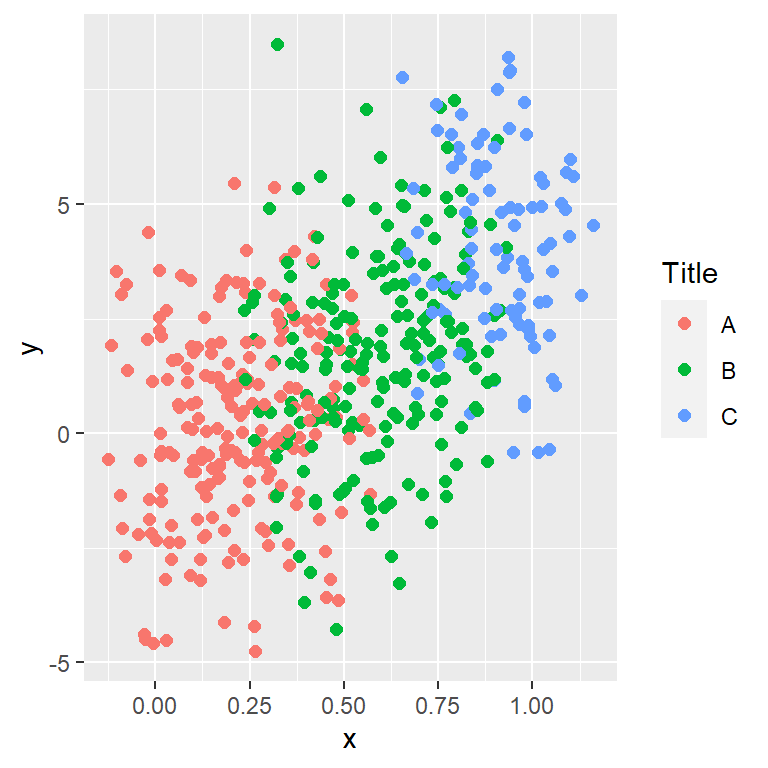Legend title

When you create a scatter plot by group in ggplot2 an automatic legend is created based con the categorical variable. The default title of the legend is the name of the variable, but you can override this with the following code.

``````# install.packages("ggplot2")
library(ggplot2)

ggplot(df, aes(x = x, y = y, color = group)) +
geom_point(size = 2) +
guides(colour = guide_legend(title = "Title"))``````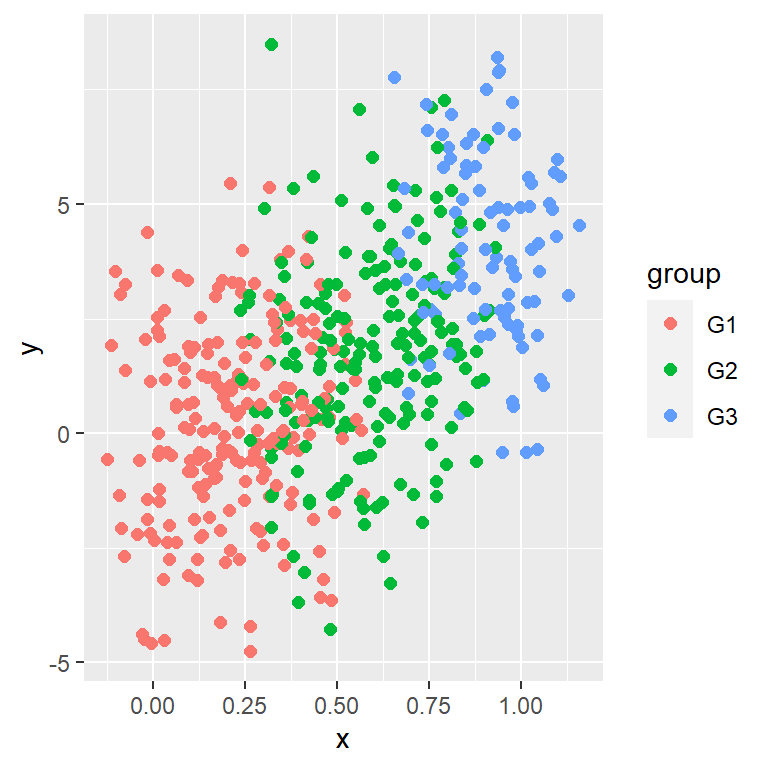Custom key labels

The labels can also be customized. You can make use of the `labels` argument of the `scale_color_discrete` function to change them.

``````# install.packages("ggplot2")
library(ggplot2)

ggplot(df, aes(x = x, y = y, color = group)) +
geom_point(size = 2) +
scale_color_discrete(labels = c("G1", "G2", "G3"))``````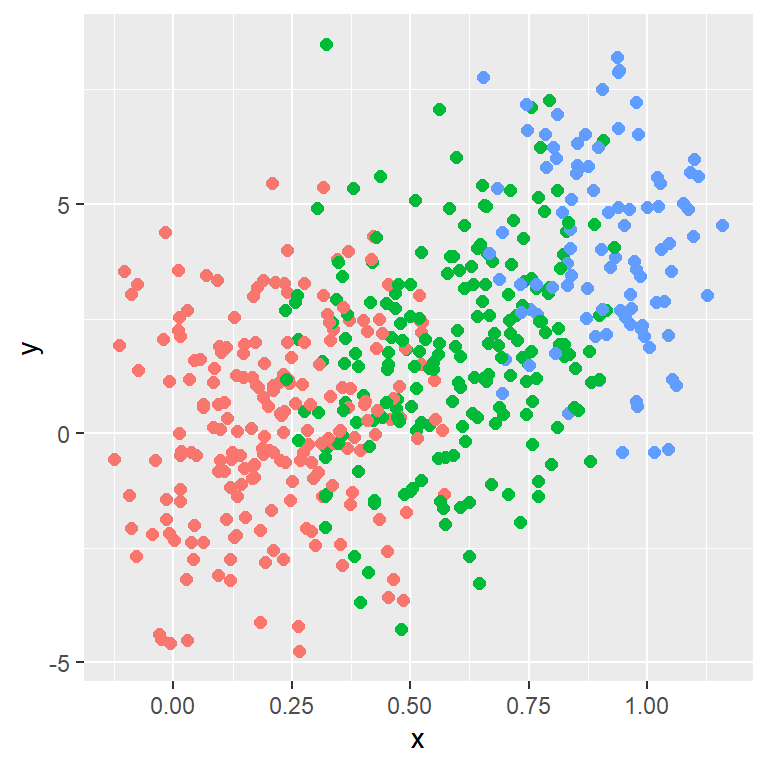Remove the legend

In case you want to get rid of the legend set `legend.position = "none"` inside the `theme` function.

``````# install.packages("ggplot2")
library(ggplot2)

ggplot(df, aes(x = x, y = y, color = group)) +
geom_point(size = 2) +
theme(legend.position = "none")``````